# A quick introduction to machine learning#

• These notebooks are a brief, hands-on, introduction to machine learning.

• We will revise some of the nomenclature, principles, and applications from Valentina’s presentation.

## ML will solve all of our problems, right?#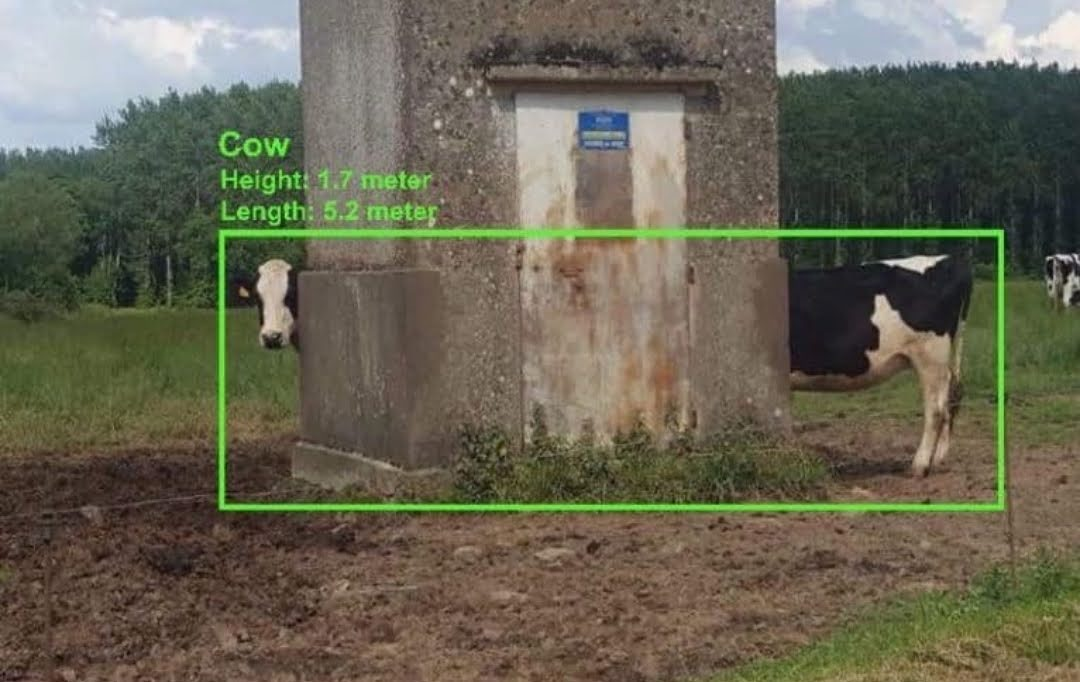## What is Machine Learning (ML)?#

Caveat: I’m not a Statistician, Mathematician, or ML expert. I only play one online. You can find my work on movies like “How to get by with little to no data” or “Oh gosh, the PI wants some buzz-words in the report” and “Fuzzy logic no longer does it, we need ML → AI → DL”

What is ML (a personal point of view):

• Focus on practical problems

• Learn from the data and/or make predictions with it

• Middle ground between statistics and optimization techniques

• We have fast computers now, right? Let them do the work! (Must see JVP talk on this.)

Oversimplified take: Fit a model to data and use it to make predictions. (This is how scikit-learn designed its API BTW).

## Vocabulary#

• parameters: Variables that define the model and control its behavior.

• model: Set of mathematical equations used to approximate the data.

• labels/classes: Quantity/category that we want to predict

• features: Observations (information) used as predictors of labels/classes.

• training: Use features and known labels/classes to fit the model estimate its parameters (full circle, right? But why stop now?).

Please check out this awesome lecture on ML for climate science.

• hyper-parameters: Variables that influence the training and the model but are not estimated during training.

• unsupervised learning: Extract information and structure from the data without training with known labels. We will see clustering, and Principal Component Analysis (PCA).

• supervised learning: Fit a model using data to “train” it for making predictions. Examples: regression, classification, spam detection, recommendation systems. We’ll see KNN, a classification type of ML in this tutorial.

## Unsupervised: PCA#

The dataset we will use was consists of Red, Green, Blue composites (parameters) from plastic pellets photos. We also have some extra information on the pellet size, shape, etc.

The labels are the yellowing index. The goal is to predict the yellowing based the pellets image, broken down to its RGB info.

```import pandas as pd
```
```df = pd.read_csv("pellets-visual-classes-rgb.csv", index_col="image").dropna()
df["yellowing index"] = df["yellowing index"].astype(int)
df
```
r g b size (mm) color description erosion erosion index yellowing yellowing index
image
cl1_p11_moca2_deixa5_a0001 152 150 143 4.021 transparent sphere high erosion 3 low 1
cl1_p12_lagoinha_deixa1_g0006 221 218 219 4.244 white light erosion low erosion 1 low 1
cl1_p12_lagoinha_deixa1_g0007 140 137 129 3.946 white not erosion low erosion 1 low 1
cl1_p12_lagoinha_deixa1_g0008 188 178 146 3.948 white moderate erosion high erosion 3 moderate 2
cl1_p12_lagoinha_deixa2_h0004 207 200 189 6.043 white light erosion low erosion 1 moderate 2
... ... ... ... ... ... ... ... ... ... ...
cl1_p6_moca2_deixa3_a0006 186 193 155 4.546 transparent cylinder moderate erosion 2 low 1
cl1_p8_moca2_deixa5_b0001 169 168 106 3.082 transparent sphere low erosion 1 low 1
cl1_p8_moca2_deixa5_b0003 191 189 152 3.932 white sphere low erosion 1 low 1
cl1_p8_moca2_deixa5_b0004 181 156 70 3.230 white sphere moderate erosion 3 moderate 2
cl1_p9_moca2_deixa5_b0001 193 192 198 3.763 transparent sphere high erosion 3 low 1

127 rows × 10 columns

```import matplotlib.pyplot as plt

def histograms():
fig, axes = plt.subplots(figsize=(11, 11), nrows=2, ncols=2)

axes = axes.ravel()

df["erosion"].value_counts().plot.barh(ax=axes, title="erosion")
df["color"].value_counts().plot.barh(ax=axes, title="color")
df["description"].value_counts().plot.barh(ax=axes, title="description")
df["yellowing"].value_counts().plot.barh(ax=axes, title="yellowing")

axes.yaxis.set_label_position("right")
axes.yaxis.tick_right()

axes.yaxis.set_label_position("right")
axes.yaxis.tick_right()
```
```histograms();
```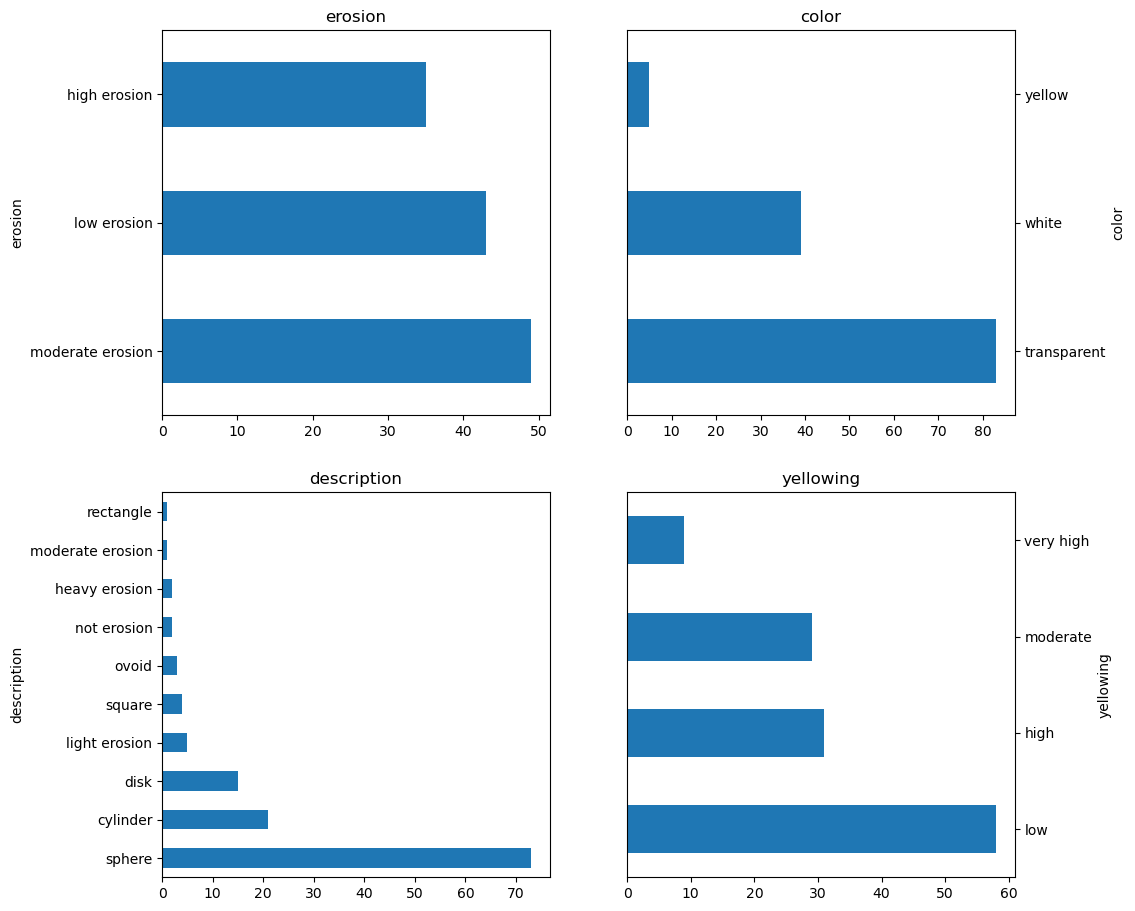We will be using only the R, G, B data for now.

```RGB = df[["r", "g", "b"]]
```
```import numpy as np
import seaborn

corr = RGB.corr()

seaborn.heatmap(corr, vmin=-1, vmax=1, annot=True);
```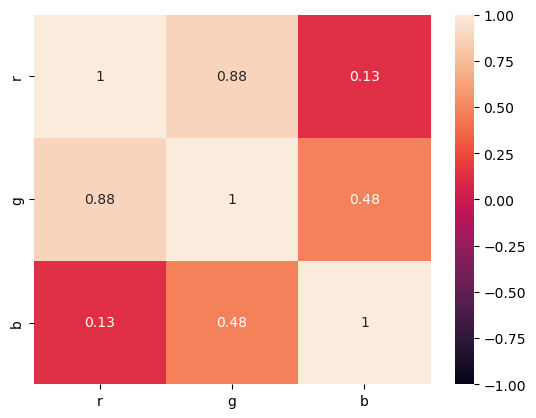The first step to most ML techniques is to standardize the data. We do not want high variance data to bias our model.

```def z_score(x):
return (x - x.mean()) / x.std()

zs = RGB.apply(z_score).T

zs.std(axis=1)  # Should be 1
```
```r    1.0
g    1.0
b    1.0
dtype: float64
```
```zs.mean(axis=1)  # Should be zero
```
```r    3.020331e-16
g    4.056248e-16
b   -1.188900e-16
dtype: float64
```
```from sklearn.decomposition import PCA

pca = PCA(n_components=None)
pca.fit(zs);
```

The pca object, or fitted model, was designed before pandas existed and it is based on numpy arrays. We can do better nowadays and add meaningful labels to it.

```loadings = pd.DataFrame(pca.components_.T)
```
TS 1 TS 2 TS 3
PC 1 0.106617 -0.020035 0.796085
PC 2 0.038558 -0.015377 0.045291
PC 3 0.118255 -0.029110 -0.071213
PC 4 0.032419 -0.036895 -0.025253
PC 5 0.037738 -0.033506 0.003937
... ... ... ...
PC 123 0.037121 0.082580 0.065741
PC 124 0.023213 0.049475 0.000340
PC 125 0.027663 0.022751 -0.036524
PC 126 -0.032039 -0.082355 0.004122
PC 127 0.076820 -0.015290 -0.064717

127 rows × 3 columns

```PCs = np.dot(loadings.values.T, RGB)
```
```marker = {
"linestyle": "none",
"marker": "o",
"markersize": 7,
"color": "blue",
"alpha": 0.5,
}

fig, ax = plt.subplots(figsize=(7, 2.75))
ax.plot(PCs, PCs, label="Scores", **marker)

ax.set_xlabel("PC1")
ax.set_ylabel("PC2")

text = [ax.text(x, y, t) for x, y, t in zip(PCs, PCs + 0.5, RGB.columns)]
```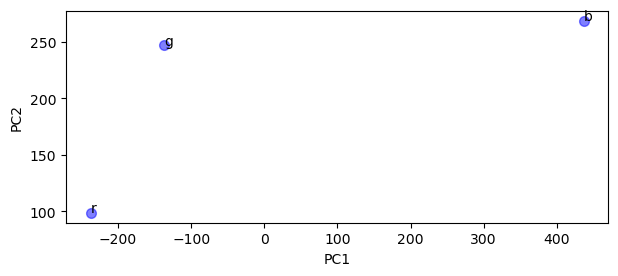```perc = pca.explained_variance_ratio_ * 100
perc = pd.DataFrame(
perc,
columns=["Percentage explained ratio"],
index=["PC %s" % pc for pc in np.arange(len(perc)) + 1],
)
ax = perc.plot(kind="bar")
```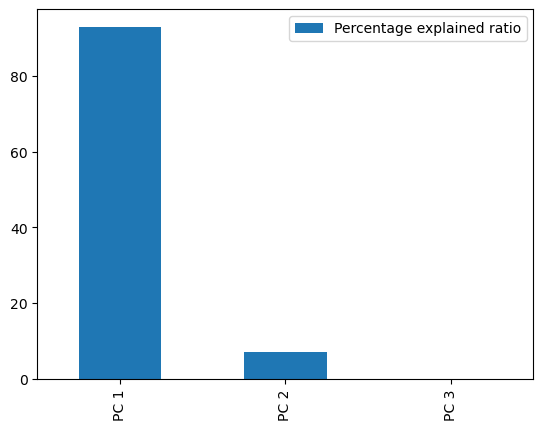The non-projected loadings plot can help us see if the data has some sort of aggregation that we can use.

```common = {"linestyle": "none", "markersize": 7, "alpha": 0.5}

markers = {
0: {"color": "black", "marker": "o", "label": "no yellowing"},
1: {"color": "red", "marker": "^", "label": "low"},
2: {"color": "blue", "marker": "*", "label": "moderate"},
3: {"color": "khaki", "marker": "s", "label": "high"},
4: {"color": "darkgoldenrod", "marker": "d", "label": "very high"},
}

fig, ax = plt.subplots(figsize=(7, 7))
for x, y, idx in zip(
):
ax.plot(x, y, **common, **markers.get(idx))

ax.set_xlabel("non-projected PC1")
ax.set_ylabel("non-projected PC2")
ax.axis([-1, 1, -1, 1])
ax.axis([-0.25, 0.25, -0.4, 0.4])

# Trick to remove duplicate labels from the for-loop.
handles, labels = ax.get_legend_handles_labels()
by_label = dict(zip(labels, handles))
ax.legend(by_label.values(), by_label.keys())
return fig, ax
```
```unprojected_loadings();
```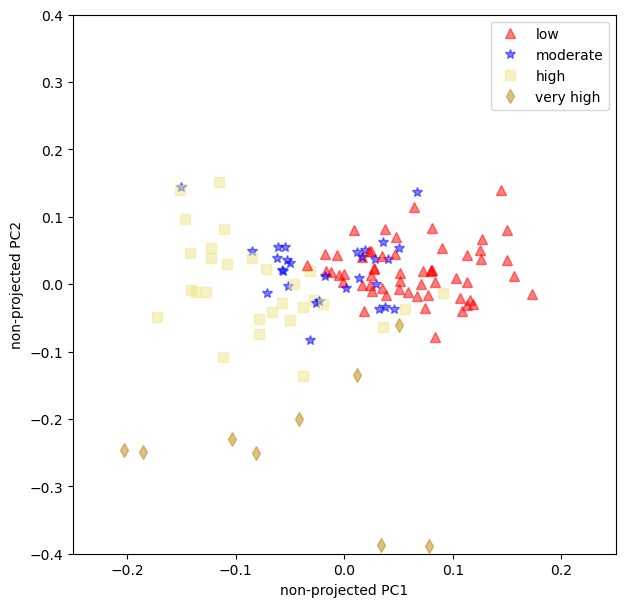## Summary#

• PCA is probably be most robust, and easy to perform, non-supervised ML technique (it has been a common technique in ocean sciences since before the ML hype);

• We learned that a single RGB value does not have enough predictive power to be used alone, we’ll need at least a combination of Reds and Greens;

• The loading plot show that the moderate and the low yellowing have some overlaps. That can be troublesome when using this model for predictions.